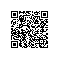# NOIP-C++大神培养计划Step1.1.1基础算法——模拟算法1

A+B Problem

#include<iostream>
using namespace std;
int main()
{
int a,b;
cin>>a>>b;
cout<<a+b<<endl;
return 0;
}

https://www.luogu.org/problemnew/show/P1003

a,b,g,k ，每两个整数之间用一个空格隔开，分别表示铺设地毯的左下角的坐标(a,b)以及地毯在x轴和y轴方向的长度第n+2行包含两个正整数x和y，表示所求的地面的点的坐标(x,y)

3
1 0 2 3
0 2 3 3
2 1 3 3
2 2

3

3
1 0 2 3
0 2 3 3
2 1 3 3
4 5

-1

【样例解释1】

1 号地毯用实线表示，
2 号地毯用虚线表示，
3 号用双实线表示，覆盖点(2,2)的最上面一张地毯是 3 号地毯。

【数据范围】

n≤2 ；

0≤a,b,g,k≤100；

0≤n≤10,000 ，
0≤a,b,g,k≤100,000。
noip2011提高组day1第1题

#include<iostream>
using namespace std;
int s,n;//二维数组用于存每一点的覆盖情况
int x,y,a,b;//地毯的大小
int X,Y;//询问坐标
int main()
{
cin>>n;
for(int i=1;i<=n;i++)
{
cin>>x>>y>>a>>b;
for(int j=x;j<=x+a;j++)//枚举X轴
{
for(int t=y;t<=y+b;t++)//枚举Y轴
{
s[j][t]=i;//二维数组赋值
}
}
}
cin>>X>>Y;
if(s[X][Y])
cout<<s[X][Y]<<endl;
else
cout<<"-1"<<endl;
return 0;
}

0≤n≤10,000 ，
0≤a,b,g,k≤100,000。

s 100001

10^6啊，很大，100000*100000是绝对不可能的。

#include<iostream>
using namespace std;
int s,n;//二维数组存x,y,a,b
int X,Y;//所求坐标
int main()
{
cin>>n;
for(int i=1;i<=n;i++)
{
cin>>s[i]>>s[i]>>s[i]>>s[i];
s[i]+=s[i];
s[i]+=s[i];
}
//s数组作用为：s[i]:第i张地毯起始点的x轴;s[i]：第i张地毯起始点的y轴;s[i]：第i张地毯终点的x轴;s[i]：第i张地毯终点的x轴
cin>>X>>Y;
for(int i=n;i>=1;i--)
{
if(s[i]<=X&&s[i]<=Y&&s[i]>=X&&s[i]>=Y)
{
cout<<i<<endl;
return 0;
}
}
cout<<-1<<endl;//没搜到输出-1.
return 0;
}

https://www.luogu.org/problemnew/show/P1008

192 384 576

...

（输出被和谐了）QAQ

#include<cstdio>
#include<cstring>
int i,j,a;
bool v;//a[i]表示第i个数已经用过了
int main()
{
for(i=192;i<=327;i++)//第一个数最小192，最大327。用数学计算的，可以减小复杂度。
{
memset(a,0,sizeof(a));
a=0;
v[i%10]=v[i/10%10]=v[i/100]=v[i*2%10]=v[i*2/10%10]=v[i*2/100]=v[i*3%10]=v[i*3/10%10]=v[i*3/100]=1;//统计数字
for(j=1;j<=9;j++)
a+=v[j];//a表示1-9这些数字是否全部齐了
if(a==9)
printf("%d %d %d\n",i,i*2,i*3);//如果齐了就输出
/*
算法介绍：
这里的i表示第一个数，上面那个很长的语句就是将3个三位数的个、十、百位统计，a来计算用了几个数，若用了9个，输出。
*/
}
return 0;
}使用钉钉扫一扫加入圈子
+ 订阅